# DFT MAGNITUDES

The DFT Example 1 results of |X(1)| = 4 and |X(2)| = 2 may puzzle the reader because our input x(n) signal, from Eq. (3-11), had peak amplitudes of 1.0 and 0.5, respectively. There's an important point to keep in mind regarding DFTs defined by Eq. (3-2). When a real input signal contains a sinewave component of peak amplitude Ao with an integral number of cycles over N input samples, the output magnitude of the DFT for that particular sinewave is Mr where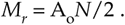If the DFT input is a complex sinusoid of magnitude Ao (i.e., Aoej2pft) with an integral number of cycles over N samples, the output magnitude of the DFT is Mc where

Equation 3-17'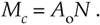As stated in relation to Eq. (3-13'), if the DFT input was riding on a DC value equal to Do, the magnitude of the DFT's X(0) output will be DoN.

Looking at the real input case for the 1000 Hz component of Eq. (3-11), Ao = 1 and N = 8, so that Mreal = 1 · 8/2 = 4, as our example shows. Equation (3-17) may not be so important when we're using software or floating-point hardware to perform DFTs, but if we're implementing the DFT with fixed-point hardware, we have to be aware that the output can be as large as N/2 times the peak value of the input. This means that, for real inputs, hardware memory registers must be able to hold values as large as N/2 times the maximum amplitude of the input sample values. We discuss DFT output magnitudes in further detail later in this chapter. The DFT magnitude expressions in Eqs. (3-17) and (3-17') are why we occasionally see the DFT defined in the literature as

Equation 3-18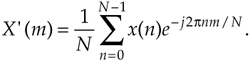The 1/N scale factor in Eq. (3-18) makes the amplitudes of X'(m) equal to half the time-domain input sinusoid's peak value at the expense of the additional division by N computation. Thus, hardware or software implementations of the DFT typically use Eq. (3-2) as opposed to Eq. (3-18). Of course, there are always exceptions. There are commercial software packages using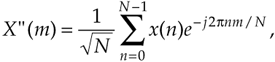and

Equation 3-18'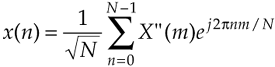for the forward and inverse DFTs. (In Section 3.7, we discuss the meaning and significance of the inverse DFT.) The 1/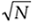scale factors in Eqs. (3-18') seem a little strange, but they're used so that there's no scale change when transforming in either direction. When analyzing signal spectra in practice, we're normally more interested in the relative magnitudes rather than absolute magnitudes of the individual DFT outputs, so scaling factors aren't usually that important to us.

URL http://proquest.safaribooksonline.com/0131089897/ch03lev1sec4AmazonPrev don't be afraid of buying books Next